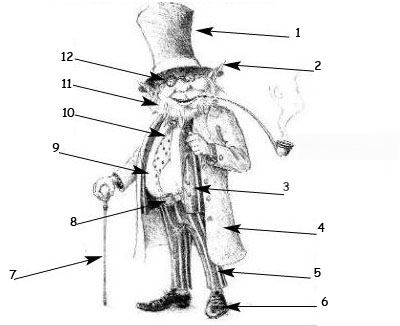## Complète la légende de ce dessin avec les mots manquants :

### by C. Chalmin

Fill in* all the gaps*, then click on "Check" to check your answers
(use the "Hint" button to get a free letter if you have problems with an answer. You can also click on the "[?]" button to get a clue. Note that you will lose points if you ask for hints or clues !)
[*to fill in = to complete / a gap = a blank space]

 12 = 11 = 10 = 9 = 8 = 7 =1 = 2 = 3 = 4 = 5 = 6 =